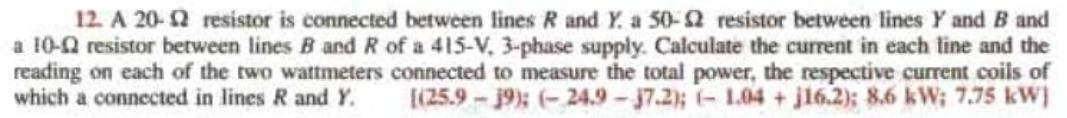Home / Expert Answers / Electrical Engineering / solve-it-handwritten-amp-correct-ans-is-mention-show-step-39-s-if-found-that-you-copy-from-ai-then-pa703

# (Solved): Solve it handwritten & Correct Ans is Mention Show Step's. If Found that You Copy From ai then ...

Solve it handwritten & Correct Ans is Mention

Show Step's.

If Found that You Copy From ai then dislike staright Forward ? & maybe Termination of your I'd.

Ai is Providing Wrong Answers12. A $$20-\Omega$$ resistor is connected between lines $$R$$ and $$Y$$ a a $$50-\Omega$$ resistor between lines $$Y$$ and $$B$$ and a 10- $$\Omega$$ resistor between lines $$B$$ and $$R$$ of a 415-V, 3-phase supply. Calculate the current in each line and the reading on each of the two wattmeters connected to measure the total power, the respective current coils of which a connected in lines $$R$$ and $$\gamma$$. $$[(25.9-j 9) ;(-24.9-j 7.2) ;(-1.04+j 16.2) ; 8.6 \mathrm{~kW} ; 7.75 \mathrm{~kW}]$$

We have an Answer from Expert